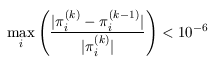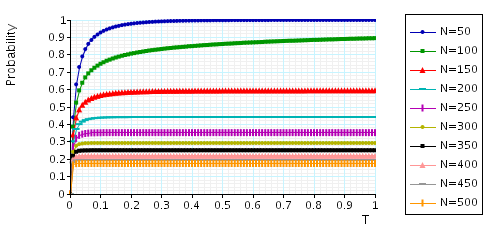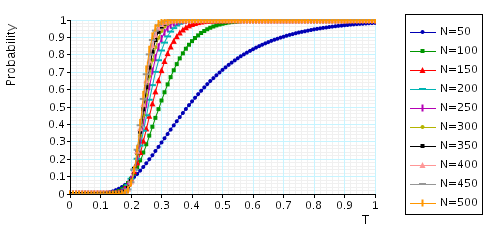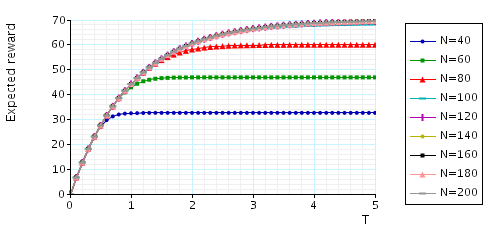# Wireless Communication Cell

(Haring, Marie, Puigjaner and Trivedi)

### Introduction

This case study is based on a single cell in a wireless communication network. It is taken from [HMPT00]. The model includes the phenomenon of hand off which we now explain.

When a mobile station moves across a cell boundary the channel in the earlier cell is released and an idle channel is required in the target cell. If an idle channel is available in the target cell the hand off is resumed and otherwise the hand off is dropped.

Since dropping of a hand off is generally considered more serious than blocking, to reduce the probability of a hand off being dropped, a guarded channel scheme (GCS) is used: a fixed number of channels (called guarded channels) are reserved exclusively for the hand off calls.

The model is represented as a CTMC. We use N to denote the number of channels in the cell and suppose that the number of guarded channel is 20% of the total number of channels. The PRISM source code for this case study is given below.

```// single cell in wireless communication network [HMPT01]
// gxn/dxp 5/3/01

ctmc

const int N; // N - number of channels
const double lambda1=49; // arrival rate of new calls
const double lambda2=21; // arrival rate of hand-off calls
const double mu=1; // departure rate of calls

module cell

n : [0..N]; // number of calls in the cell

// arrival of new call
[] (n<N*0.8) -> lambda1 : (n'=n+1);
// arrival of hand of call
[] (n<N) -> lambda2 : (n'=n+1);
// completion of call or mobile departs cell
[] (n>0) -> n*mu : (n'=n-1);

endmodule

// rewards - number calls in the cell
rewards "calls"
true : n;
endrewards
```

Extensions to this model include:

• For a fixed number of channels, vary the number of guarded channels and analyse the differences in performance.
• Consider a network of the above cells under different topologies.
• Add a control channel to a cell which is used for transmitting control messages to the system.
• Add failures and recoveries to all of the above.

### Model Statistics

The table below shows statistics for each model we have built. It gives information about the CTMC representing the model: the number of states and the number of non zeros (transitions).

 N: Model: States: NNZ: 50 51 100 100 101 200 150 151 300 200 201 400 250 251 500 300 301 600 350 351 700 400 401 800 450 451 900 500 501 1,000

Note that the above statistics are independent of the number of guarded channels.

### Model Construction Times

The table below gives the times taken to construct the models. This process involves first building a CTMC (represented as an MTBDD) from the system description (in our module based language), and then computing the reachable states using a BDD based fixpoint algorithm. The total time required and the number of fixpoint iterations are given below.

 N: Construction: Time (s): Iter.s: 50 0.058 51 100 0.093 101 150 0.128 151 200 0.139 201 250 0.188 251 300 0.269 301 350 0.245 351 400 0.296 401 450 0.345 451 500 0.425 501

### Model Checking

We now report on the model checking results obtained using PRISM when verifying the following CSL properties.

```const double T; // time bound

// the maximum probability that a hand off call can be dropped within t time units (assuming a guarded channel is free)
P=?[ true U<=T (n=N) {n<N}{max} ]

// the maximum probability that a call can be dropped within t time units (assuming a non-guarded channel is free)
P=?[ true U<=T (n>=N*0.8) {n<N*0.8}{max} ]

// the minimum probability that a cell will be accepted within T time units (assuming no channels are free)
P=?[ true U<=T (n<N*0.8) {n=N}{min} ]

// the expected number of calls at time T
R{"calls"}=? [ I=T ]

// the probability that in the long run any call can be dropped
S=? [ n<N*0.8 ]

// the expected number calls in the cell in the long run
R{"calls"}=? [ S ]

```

All experiments were carried out on a 2.0GHz PC with 512 Gb memory running Linux and in all iterative methods we stop iterating when the relative error between subsequent iteration vectors is less than 1e-6, that is:• The maximum probability that a hand off call can be dropped within t time units (assuming a guarded channel is free): the table and graph below presents the statistics and results for the model checking process.

 c: T=0.5: Iters: Time per iter: (s) MTBDD Sparse Hybrid 50 227 0.000793 < 0.00001 < 0.00001 100 247 0.002186 < 0.00001 < 0.00001 150 268 0.000370 0.000037 0.000037 200 288 0.000250 0.000035 0.000035 250 309 0.000227 0.000032 0.000065 300 335 0.000357 0.000090 0.000090 350 360 0.000741 0.000111 0.000083 400 386 0.000770 0.000155 0.000130 450 411 0.000870 0.000170 0.000146 500 437 0.000970 0.000183 0.000206• The maximum probability that a call can be dropped within t time units (assuming a non-guarded channel is free): the graph below presents the results for this property.• The minimum probability that a cell will be accepted within T time units (assuming no channels are free): the graph below presents the results for this property.• The expected number of calls in the cell at time T: the table and graph below presents the model checking statistics for this property.

 c: T=1: Iters: Time per iter: (s) MTBDD Sparse Hybrid 50 216 0.000880 < 0.00001 < 0.00001 100 551 0.001760 0.000018 < 0.00001 150 669 0.002661 < 0.00001 < 0.00001 200 790 0.003557 < 0.00001 0.000013 250 913 0.004545 0.000011 0.000011 300 1,060 0.005519 0.000009 0.000019 350 1,206 0.006650 0.000008 0.000017 400 1,351 0.007646 0.000007 0.000022 450 1,494 0.008963 0.000007 0.000027 500 1,637 0.009505 0.000012 0.000024• Lon run average properties: the table below gives the model checking statistics when computing the steady state probabilities for both the JOR and SOR methods when using PRISM's different engines.

 c: JOR: SOR: Iters: Time per iter: (s) Iters: Time per iter: (s) MTBDD Sparse Hybrid Sparse Hybrid 50 301 0.001018 < 0.00001 < 0.00001 173 < 0.00001 < 0.00001 100 1,400 0.001960 < 0.00001 < 0.00001 771 < 0.00001 0.000013 150 1,894 0.002829 0.000005 0.000011 1,139 0.000009 0.000018 200 2,254 0.003745 0.000004 0.000009 1,298 0.000015 0.000023 250 2,491 0.005122 0.000008 0.000012 1,419 0.000021 0.000028 300 2,686 0.005481 0.000007 0.000011 1,520 0.000020 0.000033 350 2,859 0.005726 0.000010 0.000017 1,611 0.000019 0.000037 400 3,020 0.005915 0.000013 0.000017 1,696 0.000024 0.000041 450 3,171 0.006346 0.000013 0.000019 1,776 0.000028 0.000056 500 3,316 0.008201 0.000015 0.000021 1,854 0.000032 0.000054

In the graphs below we have plotted the result for the probability that in the long run any call can be dropped and the expected number of calls in the cell in the long run.